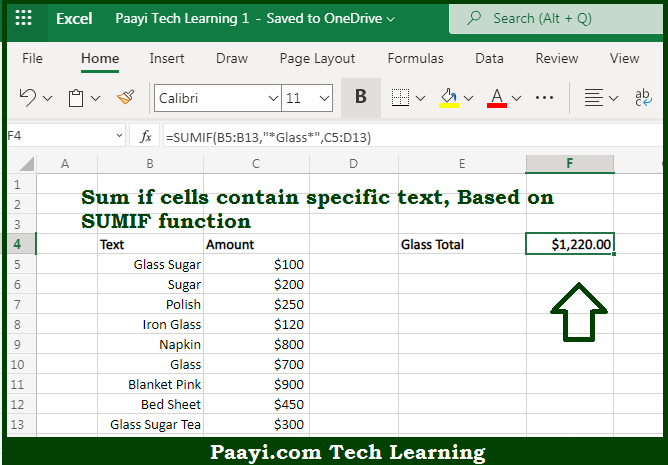# Learn How to SUM If Cell Specific Text in Microsoft Excel

Written by | 0 Comments | 587 Views

In this article, you will learn how to SUM various things in Microsoft Excel using a single/combination(s) of functions. You will also know how to SUM If Cell-Specific Text and see the generic formula.

SUM If Cell-Specific Text in Microsoft Excel

The main purpose of this formula is to sum if cells contain specific text. Here we will learn how to sum if cells is having any specific text string in the workbook in Microsoft Excel. That implies, with the help of a formula based on the SUMIF function with wildcards you can able to sum if cells contain specific text. So, with the help of this formula, you can able to sum if cells is having any specific text string in the workbook in Microsoft Excel.

General Formula to SUM If Cell-Specific Text

=SUMIF(range,"*text*",sum_range)

The Explanation for the SUM If Cell Specific TextSo we know that with the help of the given formula above you can able to sum if cells contain specific text. Here we will learn how to sum if cells is having any specific text string in the workbook in Microsoft Excel. As we know that the SUMIF function fully supports wildcards. where the asterisk (*) means "one or more characters", while a question mark (?) means "any one character". So, with the help of this formula, you can able to sum if cells is having any specific text string in the workbook in Microsoft Excel.